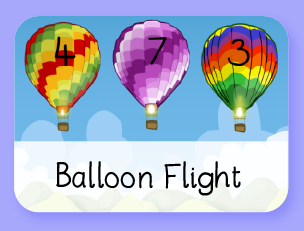# Ten Frame

### A 'concrete' model for visualising numbers up to 20.

Learning Objectives:
• YR: Children count reliably with numbers from one to 20, [...] they add and subtract two single-digit numbers and count on or back to find the answer...
• Y1: To represent and use number bonds and related subtraction facts within 20.
• Y1: To solve one-step problems that involve addition and subtraction, using concrete objects and pictorial representations, and missing number problems such as 7 = ? – 9.
A simple model for visualising numbers up to 20. Ideal for '5 and a bit'-style mental visualisations of number.

### Instructions:

You can drag on green, red or white counters. They can be moved to different cells.
The three lines allow you to fill the rest of the cells. This is to prevent teaching delays while you drag on the counters.
To remove a counter simply drag it off the frame.
You can choose from a ten frame, a 20 frame (vertical, for place value) and a 20 frame horizontal

### Examples of use:

Place 8 white counters and 2 red counters in a 10 frame. Ask the children to write as many maths sentences as they can,
8+2=10
2+8=10
10-2=8
10-8=2
5+3+2=10...

Select the vertical 20 frame.
Fill the left-hand frame. Place different numbers of counters in the right-hand frame. Model place value as you create different 'teen numbers'.
Model bonds for 20 e.g. 14 + 6= 20, by using the facts learnt for the bonds for 10. Have fun!

Build on the learning with
these games:Made using theandlibraries.